# 面试系列 -3 限流场景实践### 2 什么是接口限流PS：缓存和降级并不是能解决所有大流量情况。比如之前我做电商，千万级别的用户瞬间秒杀下单，大量的写库操作是我们无法控制的，db 的吞吐量是有瓶颈的。所以服务引入限流操作，就会大大降低服务的崩溃问题。

### 3 四种限流方案

#### 3.1 计数器算法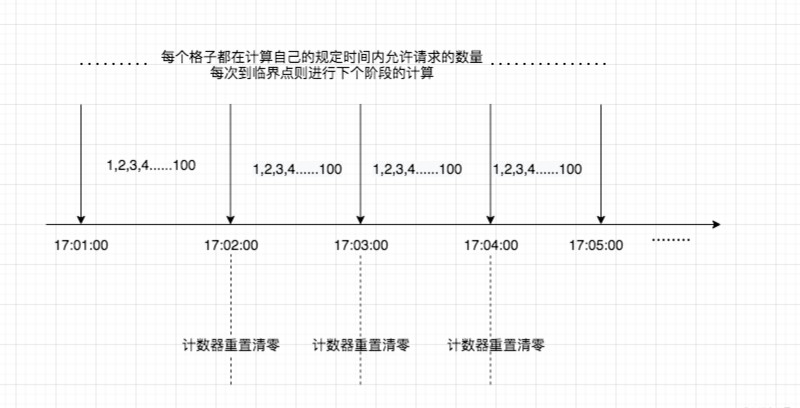/** * @desc 计数器限流 * @return bool */public function counter(){    $curr_time = time(); // 如果当前请求时间大于第一次请求时间+最大限制时间 if ($this->first_request_time + $this->fix_time >$curr_time) {        //若当前的请求数量 大于等于 限制的总数量        if ($this->request_count >=$this->request_limit) return false;        $this->request_count++; return true; } else { // 重置第一次请求时间 和 请求总次数$this->first_request_time = $curr_time;$this->request_count = 1;        return true;    }}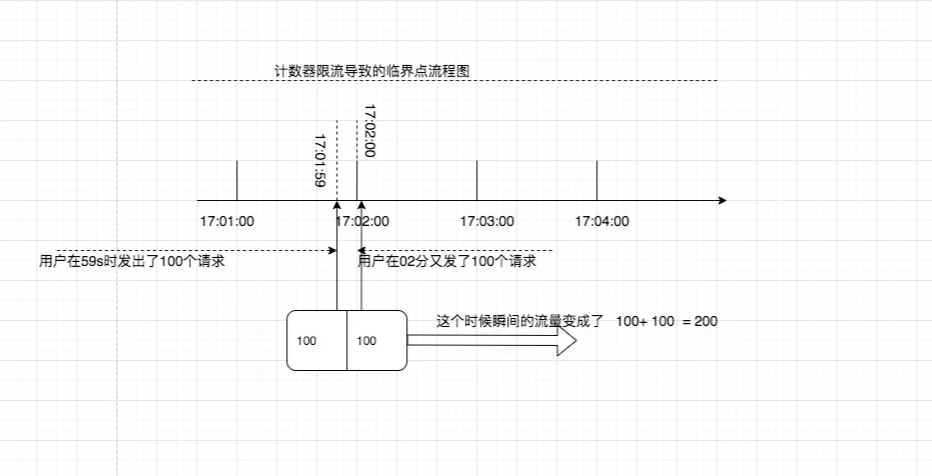#### 3.2 滑动窗口算法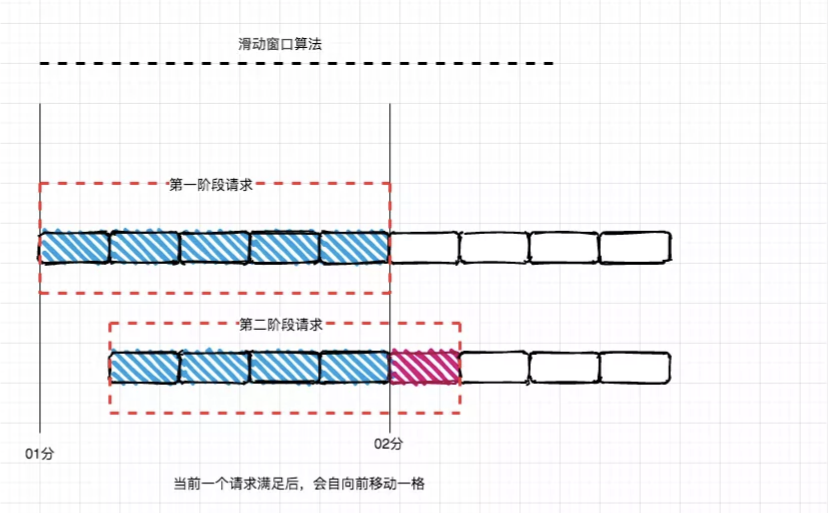/** * @desc 平滑移动方法 * @return bool */public function slide(){    $key = 'slide:test:key:user';$redis = new \Redis();    $now_time = time(); // 事务处理 按照先后顺序把命令放进一个队列当中$redis->multi();    // value + score 都使用毫秒时间戳    $redis->zAdd($key, $now_time,$now_time);    // 移除时间窗口之前的请求记录，剩下的就全部都是时间窗口内的    $redis->zRemRangeByScore($key, 0, $now_time -$this->fix_time);    // 获取窗口内的记录数量    $redis->zCard($key);    // 设置 移动窗口的过期时间 避免占用过多的内存 过期时间等于最大窗口长度 额外补加1s    $redis->expire($key, $this->fix_time + 1); // 批量执行 此操作是原子性的$result = $redis->exec();$current_count = isset($result) ?$result:0;    return $current_count <$this->request_limit;}

#### 3.3 漏桶算法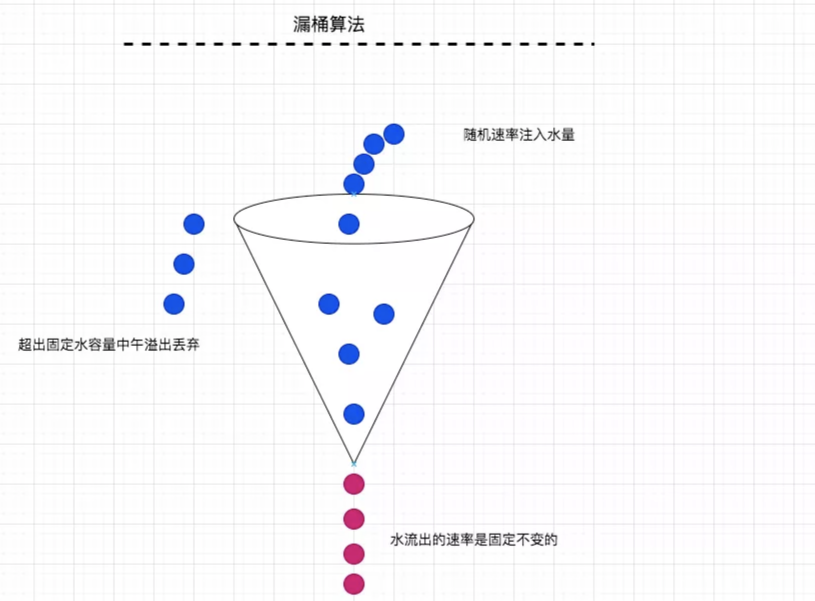/** * @desc 漏桶算法 * @return bool */public function leaky(){    // 当前请求时间戳    $curr_time = time(); // 计算当前请求的使用水量$out_water = ($curr_time -$this->last_request_time) * $this->rate; // 计算漏斗内的水量剩余多少$this->water = max(0, $this->water -$out_water);    $this->last_req_time =$curr_time;    // 若桶内水量还没有满 则往桶内继续加水    if ($this->water <$this->capacity) {        $this->water =$this->water + 1;    }    // 抱歉水桶已经满了，拒绝加水量    return false;}

#### 3.4 令牌桶算法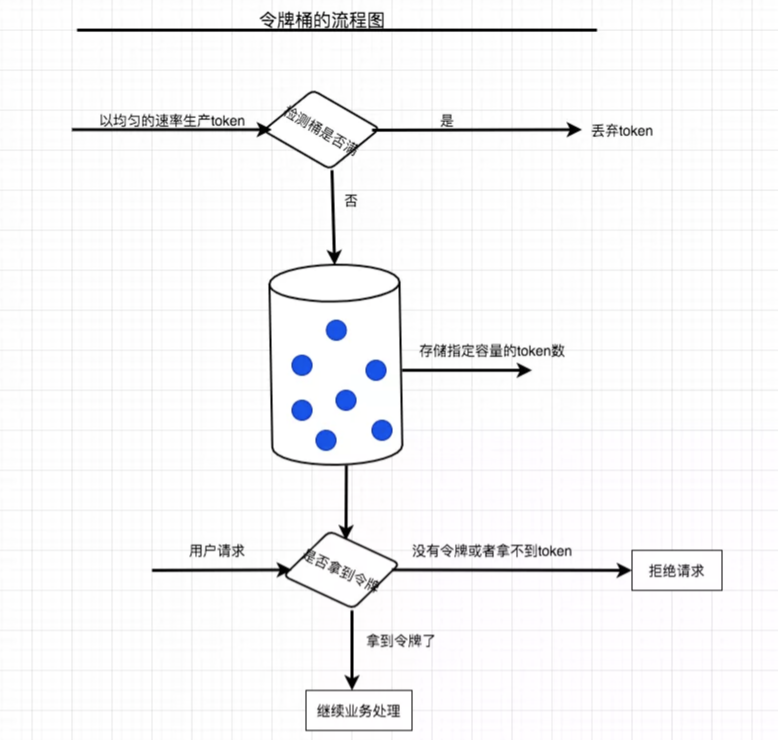/** * @desc 令牌桶方法 * @return bool */public function token(){    // 当前请求时间戳    $curr_time = time(); // 计算当前生产的令牌数$inside_token = ($curr_time -$this->last_request_time) * $this->create_token_rate; // 计算桶内还能使用的令牌数量$this->tokens = min($this->capacity_size,$this->tokens +  $inside_token);$this->last_req_time = $curr_time; // 假如桶内的令牌数少于1个 则拒绝获取 if ($this->tokens < 1) return  false;    // 令牌数还有 则移除一个    $this->tokens =$this->tokens - 1;    return true;}

/** * @desc 网令牌桶内添加令牌操作 * @param int $num 添加的数量 * @return int */public function insert($num = 0){    // 当前桶内令牌剩余数量    $curr_count = intval($this->redis->lLen($this->queue_name)); // 计算当前桶内最大可添加多少令牌 若大于桶内令牌 则相减获取应添加数量 否则直接添加$num = $this->max_volume >=$curr_count + $num ?$num : $this->max_volume -$curr_count;    // 若不能添加令牌 则返回添加0个元素    if ($num <= 0) return 0; //添加令牌操作 生成令牌数据$tokens = array_fill(0, $num, 1); // 批量添加令牌进入队列$result = $this->redis->lPush($this->queue_name, ...$tokens); if ($result) return \$num;    return 0;}

PS：以上则是实现限流的集中方案的部分代码，详细代码：https://github.com/woshiamu/amu/tree/master/redis

### 最后总结## 评论